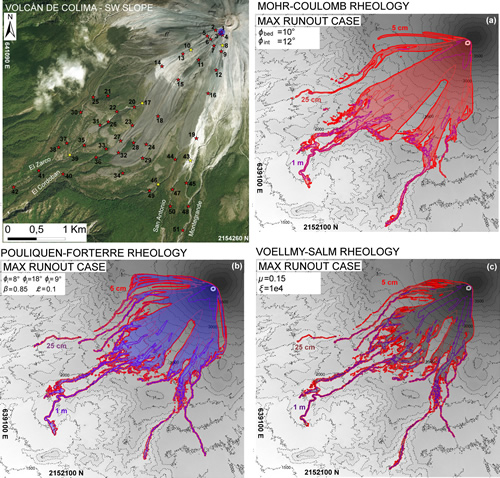Sei qui: Home > Archiviati > Comparative analysis of the structures and outcomes of geophysical flow models and modeling assumptions using uncertainty quantification

## Comparative analysis of the structures and outcomes of geophysical flow models and modeling assumptions using uncertainty quantificationFigure 2. Volcán de Colima—comparison between max flow height maps of simulated flow, assuming MC (a,d), PF (b,e), and VS (c,f) models. Extreme cases—(a–c) max. volume–min. resistance and (d–f) min. volume–max. resistance.

Patra A.K., A. Bevilacqua, A. Akhavan-Safaei, E.B. Pitman, M.I. Bursik, and D. Hyman (2020).
Frontiers in Earth Science, doi: 10.3389/feart.2020.00275.

### Abstract

We advocate here a methodology for characterizing models of geophysical flows and the modeling assumptions they represent, using a statistical approach over the full range of applicability of the models. Such a characterization may then be used to decide the appropriateness of a model and modeling assumption for use. We present our method by comparing three different models arising from different rheology assumptions, and the output data show unambiguously the performance of the models across a wide range of possible flow regimes. This comparison is facilitated by the recent development of the new release of our TITAN2D mass flow code that allows choice of multiple rheologies The quantitative and probabilistic analysis of contributions from different modeling assumptions in the models is particularly illustrative of the impact of the assumptions. Knowledge of which assumptions dominate, and, by how much, is illustrated in the topography on the SW slope of Volcán de Colima (MX). A simple model performance evaluation completes the presentation.